Science >> Physics for Kids

# Physics for Kids

## Resistors in Series and Parallel

When resistors are used in electronic circuits they can be used in different configurations. You can calculate the resistance for the circuit, or a portion of the circuit, by determining which resistors are in series and which are in parallel. We'll describe how to do this below. Note that the total resistance of a circuit is often called the equivalent resistance.

Series Resistors

When resistors are connected end-to-end in a circuit (like shown in the picture below) they are said to be in "series." In order to find the total resistance of resistors in series you just add up the value of each resistor. In the example below the total resistance would be R1 + R2.Here is another example of a number of resistors in series. The total value of the resistance across the voltage V is R1 + R2 + R3 + R4 + R5.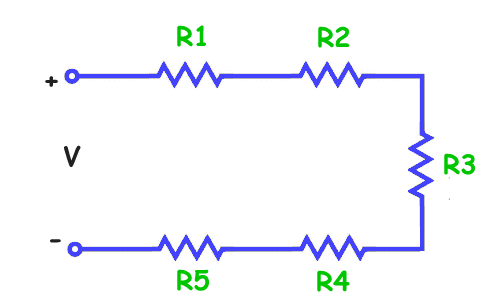Sample problem:

Using the circuit diagram below, solve for the value of the missing resistance R.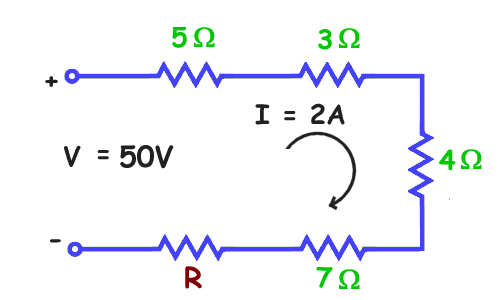First we'll figure out the equivalent resistance of the entire circuit. From Ohm's law we know that Resistance = Voltage/current, therefore

Resistance = 50volts/2amps
Resistance = 25

We can also figure out the resistance by adding up the resistors in series:

Resistance = 5 + 3 + 4 + 7 + R
Resistance = 19 + R

Now we plug in 25 for resistance and we get

25 = 19 + R
R = 6 ohms

Parallel Resistors

Parallel resistors are resistors that are connected across from each other in an electric circuit. See the picture below. In this picture R1, R2, and R3 are all connected in parallel to each other.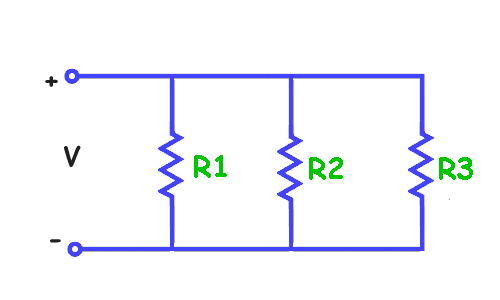When we calculated the series resistance, we totaled the resistance of each resistor to get the value. This makes sense because the current of a voltage across the resistors will travel evenly across each resistor. When the resistors are in parallel this is not the case. Some of the current will travel through R1, some through R2, and some through R3. Each resistor provides an additional path for the current to travel.

In order to calculate the total resistance "R" across the voltage V we use the following formula:You can see that the reciprocal of the total resistance is the sum of the reciprocal of each resistance in parallel.

Example problem:

What is the total resistance "R" across the voltage V in the circuit below?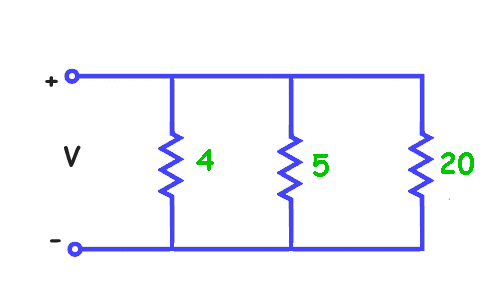Since these resistors are in parallel we know from the equation above that
1/R = ¼ + 1/5 + 1/20
1/R = 5/20 + 4/20 + 1/20
1/R = 10/20 = ½
R = 2 Ohms

Note that the total resistance is less than any of the resistors in parallel. This will always be the case. The equivalent resistance will always be less than the smallest resistor in parallel.

Series and Parallel

What do you do when you have a circuit with both parallel and series resistors?

The idea for solving these types of circuits is to break down smaller parts of the circuit into series and parallel sections. First do any sections that have only series resistors. Then replace those with the equivalent resistance. Next solve the parallel sections. Now replace those with equivalent resistors. Continue through these steps until you reach the solution.

Example problem:

Solve for the equivalent resistance across the voltage V in the electrical circuit below: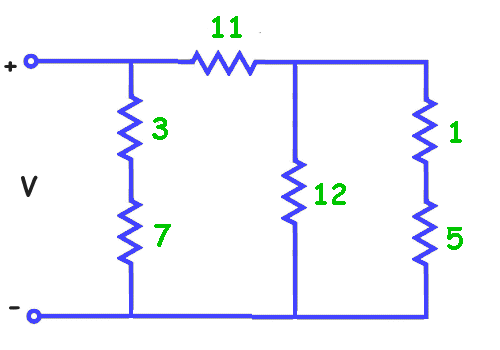First we will total the two series resistors on the right (1 + 5 = 6) and on the left (3 + 7 = 10). Now we have reduced the circuit.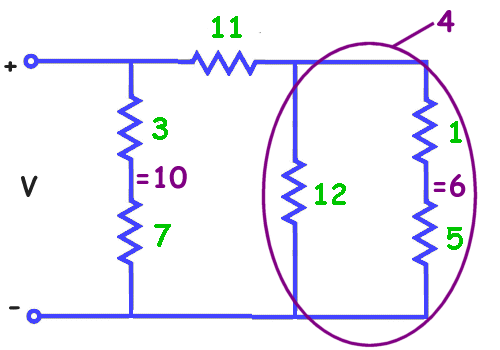We see on the right that the total resistance 6 and the resistor 12 are now in parallel. We can solve for these parallel resistors to get the equivalent resistance of 4.

1/R = 1/6 + 1/12
1/R = 2/12 + 1/12
1/R = 3/12 = ¼
R = 4

The new circuit diagram is shown below.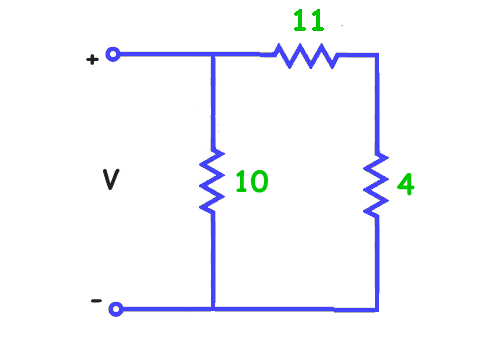From this circuit we solve for the series resistors 4 and 11 to get 4 + 11 = 15. Now we have two parallel resistors, 15 and 10.

1/R = 1/15 + 1/10
1/R = 2/30 + 3/30
1/R = 5/30 = 1/6
R = 6

The equivalent resistance across V is 6 ohms.

Activities

More Electricity Subjects

Science >> Physics for Kids